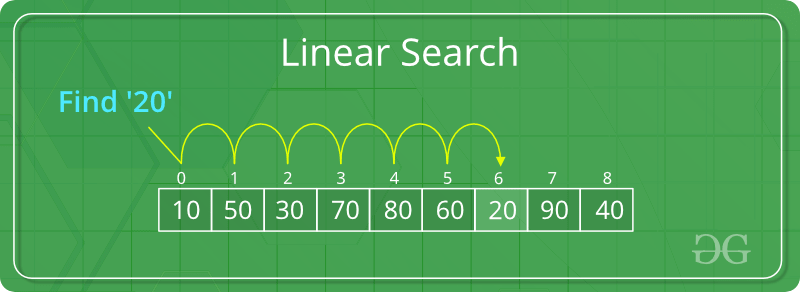Open in App
Not now

# C++ Program For Linear Search

• Last Updated : 20 Feb, 2023

Problem: Given an array arr[] of n elements, write a function to search a given element x in arr[].

Examples:

```Input: arr[] = {10, 20, 80, 30, 60, 50,
110, 100, 130, 170}
x = 110;
Output: 6
Element x is present at index 6
Input: arr[] = {10, 20, 80, 30, 60, 50,
110, 100, 130, 170}
x = 175;
Output: -1
Element x is not present in arr[].```
Recommended Problem

A simple approach is to do a linear search, i.e

• Start from the leftmost element of arr[] and one by one compare x with each element of arr[]
• If x matches with an element, return the index.
• If x doesn’t match with any of elements, return -1.Example:

## C++

 `// C++ code to linearly search ` `// x in arr[]. If x is present ` `// then return its location, ` `// otherwise return -1` `#include ` `using` `namespace` `std;`   `int` `search(``int` `arr[], ` `           ``int` `n, ``int` `x)` `{` `    ``int` `i;` `    ``for` `(i = 0; i < n; i++)` `        ``if` `(arr[i] == x)` `            ``return` `i;` `    ``return` `-1;` `}`   `// Driver code` `int` `main(``void``)` `{` `    ``int` `arr[] = {2, 3, 4, 10, 40};` `    ``int` `x = 10;` `    ``int` `n = ``sizeof``(arr) / ``sizeof``(arr);` `  `  `    ``// Function call` `    ``int` `result = search(arr, n, x);` `    ``(result == -1) ? ` `     ``cout << ``"Element is not present in array"` `: ` `     ``cout << ``"Element is present at index "` `<< ` `              ``result;` `    ``return` `0;` `}`

Output

`Element is present at index 3`

The time complexity of the above algorithm is O(n).

The space complexity of the above algorithm is O(1) as no extra space is used.

Linear search is rarely used practically because other search algorithms such as the binary search algorithm and hash tables allow significantly faster-searching comparison to Linear search.

Improve Linear Search Worst-Case Complexity

1. if element Found at last  O(n) to O(1)
2. It is the same as previous method because here we are performing 2 ‘if’ operations in one iteration of the loop and in previous method we performed only 1 ‘if’ operation. This makes both the time complexities same.

Below is the implementation:

## C++14

 `// C++ program for linear search` `#include` `using` `namespace` `std;`   `void` `search(vector<``int``> arr, ` `            ``int` `search_Element)` `{` `    ``int` `left = 0;` `    ``int` `length = arr.size();` `    ``int` `position = -1;` `      ``int` `right = length - 1;` `      `  `    ``// Run loop from 0 to right` `    ``for``(left = 0; left <= right;) ` `    ``{` `        `  `        ``// If search_element is found with` `        ``// left variable` `        ``if` `(arr[left] == search_Element) ` `        ``{` `            `  `            ``position = left;` `            ``cout << ``"Element found in Array at "` `<< ` `                     ``position + 1 << ``" Position with "` `<< ` `                     ``left + 1 << ``" Attempt"``;` `               `  `            ``break``;` `        ``}` `      `  `        ``// If search_element is found with` `        ``// right variable` `        ``if` `(arr[right] == search_Element) ` `        ``{` `            ``position = right;` `            ``cout << ``"Element found in Array at "` `<< ` `                     ``position + 1 << ``" Position with "` `<< ` `                     ``length - right << ``" Attempt"``;` `               `  `            ``break``;` `        ``}` `        ``left++;` `        ``right--;` `    ``}`   `    ``// If element not found` `    ``if` `(position == -1)` `        ``cout << ``"Not found in Array with "` `             ``<< left << ``" Attempt"``;` `}`   `// Driver code` `int` `main()` `{` `    ``vector<``int``> arr{1, 2, 3, 4, 5};` `    ``int` `search_element = 5;` `    `  `    ``// Function call` `    ``search(arr, search_element);` `}` `    `  `// This code is contributed by mayanktyagi1709`

Output

`Element found in Array at 5 Position with 1 Attempt`

Time Complexity : O(n)

Auxiliary Space: O(1)

My Personal Notes arrow_drop_up
Related Articles